Courses

# Concentrated Moment Civil Engineering (CE) Notes | EduRev

## Civil Engineering (CE) : Concentrated Moment Civil Engineering (CE) Notes | EduRev

The document Concentrated Moment Civil Engineering (CE) Notes | EduRev is a part of the Civil Engineering (CE) Course Advanced Solid Mechanics - Notes, Videos, MCQs & PPTs.
All you need of Civil Engineering (CE) at this link: Civil Engineering (CE)

Example 2: Concentrated moment

The concentrated moment, Mo, is considered to be equivalent to the action of two concentrated forces, P, equal in magnitude but opposite in direction and separated by a distance L as shown in the figure 11.5. Thus,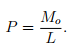(11.26)

We obtain the solution to this loading case by superposing the displacement field obtained in the above example for a single point load. Thus, it follows from equations (11.17) and (11.19) that the displacement due to the downward acting force at a distance, L/2 from the origin is,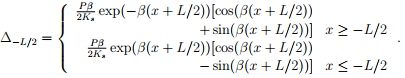(11.27)

Similarly, the displacement due to the upward acting force at a distance, −L/2 from the origin is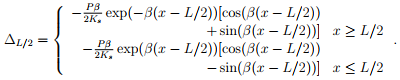(11.28)

Since, the displacement is small and the material obeys Hooke’s law, we can superpose the solutions as discussed in section 7.5.2. Hence, the displacement under the action of both the forces, ∆ = ∆L/2 + ∆−L/2 evaluates to,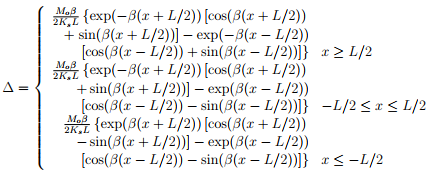(11.29)

where we have used equation (11.26). When L → 0 and P L → Mo the above equation (11.29) evaluates to,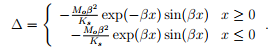(11.30)

Having found the displacement, (11.30), the variation of the bending moment along the axis of the beam obtained from (11.2) is: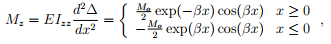(11.31)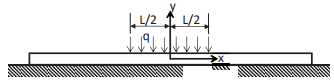Figure 11.6: Schematic of a long beam on elastic foundation subjected to uniformly distributed load of length L on either side of the mid span

and the shear force variation computed using (11.14) is: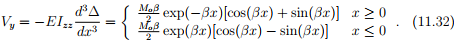Offer running on EduRev: Apply code STAYHOME200 to get INR 200 off on our premium plan EduRev Infinity!

,

,

,

,

,

,

,

,

,

,

,

,

,

,

,

,

,

,

,

,

,

;